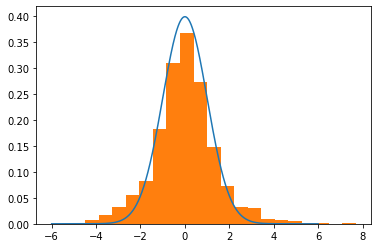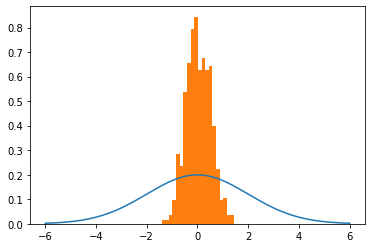# #StackBounty: #bayesian #mcmc #differential-equations #hmc How does Hamiltonian Monte Carlo work?

### Bounty: 50

I made the below graphic to explain how I currently understand the HMC algorithm. I’d like verification from a subject matter expert if this understanding is or isn’t correct. The text in the below slide is copied below for ease of access:

Hamiltonian Monte Carlo: A satellite orbits a planet. The closer the satellite is to the planet, the greater the effects of gravity. This means, (A) higher potential energy and (B) higher kinetic energy needed to sustain orbit. That same kinetic energy at a further distance from the planet, would eject the satellite out from orbit. The satellite is tasked with collecting photos of a specific geographic region. The closer the satellite orbits the planet, the faster it moves in orbit, the more times it passes over the region, the more photographs it collects. Conversely, the further a satellite is from the planet, the slower it moves in orbit, the less times it passes over the region, the less photographs it collects.
In the context of sampling, distance from the planet represents distance from the expectation of the distribution. An area of low likelihood is far from the expectation; when “orbiting this likelihood,” lower kinetic energy means less samples collected over a fixed interval of time, whereas when orbiting a higher likelihood means more samples collected given the same fixed time interval.
In a given orbit, the total energy, kinetic and potential, is constant; however, the relationship between the two is not simple. Hamiltonian equations relate changes in one to the other. Namely, the gradient of position with respect to time equals momentum. And the gradient of momentum with respect to time equals the gradient of potential energy with respect to position. To compute how far a satellite will have traveled along its orbital path, leapfrog integration must be used, iteratively updating momentum and position vectors. In the context of sampling, likelihood is analogous to distance from the planet and the gradient of potential energy with respect to position is the gradient of the probability density function with respect to its input parameter, x. This information allows the orbital path around various inputs, X, corresponding to the same likelihood, y, to be explored.
However, we’re not simply interested in exploring one likelihood, we must explore multiple orbital paths. To accomplish this, the momentum must randomly be augmented, bringing the satellite closer or further away from the planet. These random “momentum kicks” allow for different likelihoods to be orbited. Fortunately, hamiltonian equations ensure that no matter the likelihood, the number of samples collected is proportionate to the likelihood, thus samples collected follow the shape of the target distribution.

My question is – Is this an accurate way to think about how Hamiltonian Monte Carlo works?Edit:

I’ve implemented in some code based on my understanding of the algorithm. It works for a gaussian with mu=0, sigma=1. But if I change sigma it breaks. Any insights would be appreciated.

``````import numpy as np
import random
import scipy.stats as st
import matplotlib.pyplot as plt

def normal(x,mu,sigma):
numerator = np.exp((-(x-mu)**2)/(2*sigma**2))
denominator = sigma * np.sqrt(2*np.pi)
return numerator/denominator

def neg_log_prob(x,mu,sigma):
num = np.exp(-1*((x-mu)**2)/2*sigma**2)
den = sigma*np.sqrt(np.pi*2)
return -1*np.log(num/den)

def HMC(mu=0.0,sigma=1.0,path_len=1,step_size=0.25,initial_position=0.0,epochs=1_000):
# setup
steps = int(path_len/step_size) -1 # path_len and step_size are tricky parameters to tune...
samples = [initial_position]
momentum_dist = st.norm(0, 1)
# generate samples
for e in range(epochs):
q0 = np.copy(samples[-1])
q1 = np.copy(q0)
p0 = momentum_dist.rvs()
p1 = np.copy(p0)
dVdQ = -1*(q0-mu)/(sigma**2) # gradient of PDF wrt position (q0) aka momentum wrt position

# leapfrog integration begin
for s in range(steps):
p1 += step_size*dVdQ/2 # as potential energy increases, kinetic energy decreases
q1 += step_size*p1 # position increases as function of momentum
p1 += step_size*dVdQ/2 # second half "leapfrog" update to momentum
# leapfrog integration end
p1 = -1*p1 #flip momentum for reversibility

#metropolis acceptance
q0_nlp = neg_log_prob(x=q0,mu=mu,sigma=sigma)
q1_nlp = neg_log_prob(x=q1,mu=mu,sigma=sigma)

p0_nlp = neg_log_prob(x=p0,mu=0,sigma=1)
p1_nlp = neg_log_prob(x=p1,mu=0,sigma=1)

# Account for negatives AND log(probabiltiies)...
target = q0_nlp - q1_nlp # P(q1)/P(q0)
adjustment = p1_nlp - p0_nlp # P(p1)/P(p0)

event = np.log(random.uniform(0,1))
if event <= acceptance:
samples.append(q1)
else:
samples.append(q0)

return samples
``````

Now it works here:

``````mu, sigma = 0,1
trial = HMC(mu=mu,sigma=sigma,path_len=2,step_size=0.25)

# What the dist should looks like
lines = np.linspace(-6,6,10_000)
normal_curve = [normal(x=l,mu=mu,sigma=sigma) for l in lines]

# Visualize
plt.plot(lines,normal_curve)
plt.hist(trial,density=True,bins=20)
plt.show()
``````But it breaks when I change sigma to 2.

``````# Generate samples
mu, sigma = 0,2
trial = HMC(mu=mu,sigma=sigma,path_len=2,step_size=0.25)

# What the dist should looks like
lines = np.linspace(-6,6,10_000)
normal_curve = [normal(x=l,mu=mu,sigma=sigma) for l in lines]

# Visualize
plt.plot(lines,normal_curve)
plt.hist(trial,density=True,bins=20)
plt.show()
``````Any ideas? I feel like I’m close to "getting it".

Get this bounty!!!

This site uses Akismet to reduce spam. Learn how your comment data is processed.